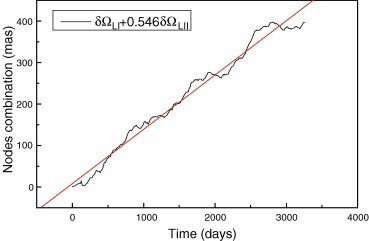# Circling the Drain

In Relativity by Brian Koberlein4 Comments

If you’ve ever watched water drain from a bathtub, you’ve seen that it doesn’t flow into the drain in a straight line, but rather swirls around the drain.  Contrary to popular belief, this is not due to the rotation of the Earth but rather random currents in the water.  The reason the spiral forms is because it is self-reinforcing. Water near the drain spirals a bit due to a random current, which drags the water behind it slightly in the same direction.  Over time, the motion builds up until you have a rapid spiral around the drain.

There is a similar effect with space and time.  I’ve talked before about how gravity is due to a curvature of space and time. The presence of mass bends space around it, and the resulting curvature means that objects follow curved paths rather than straight ones.  As a result, an object’s motion near a large mass like the Earth looks as if it is due to a force, which we call gravity.

It turns out that the rotation of a mass also distorts space and time.  For example, as the Earth rotates, it drags the nearby space along with it (an effect known as frame dragging).  Just like the drain spiral, this effect builds up, and as a result, space spirals a bit around the Earth.  You have to be a bit careful with this comparison.  Spacetime doesn’t “flow” the way water does, but the spiral effect is somewhat similar.

Near the Earth, this frame dragging is very small, but it can be measured through an effect known as the Lense-Thirring effect.  Basically, you put a gyroscope in orbit and see if its axis of rotation changes.  If there is no frame dragging, then the orientation of the gyroscope shouldn’t change.  If there is frame dragging, then the spiral twist of space and time will cause the gyroscope to precess, and its orientation will slowly change over time.

We’ve actually done this experiment, and you can see the results in the figure below.  The black line represents the change in orientation over time, and the red line is the predicted change via the Lense-Thirring effect.  As you can see, they agree very well.So the next time you watch water circling the drain, you will know that a similar effect occurs with space and time itself.

1.Do you know why there is max in measurements roughly every 1000 days?

1.Space has to wind to some local maximum of curvature, and then snap back. Since Earth’s mass is constant, this maximum winding should be predictable.

2.What i think is that , by the time you put the gyroscope in orbit there is a max distance and a min distance from earth (supposing that orbit is an elipse) . So if the period is almost 1000 days then every 1000 days it will reach the min distance from earth where the gravity field is max so the frame dragging should be max .

2.If the water spiralling down the drain is just due to “random currents in the water” and not the Earths rotation, why is the spiral always the same direction of rotation? If it was truly random, surely it would be 50/50 on direction of rotation?